Courses

# Code Generation & Optimization - 2

## 15 Questions MCQ Test Question Bank for GATE Computer Science Engineering | Code Generation & Optimization - 2

Description
This mock test of Code Generation & Optimization - 2 for Computer Science Engineering (CSE) helps you for every Computer Science Engineering (CSE) entrance exam. This contains 15 Multiple Choice Questions for Computer Science Engineering (CSE) Code Generation & Optimization - 2 (mcq) to study with solutions a complete question bank. The solved questions answers in this Code Generation & Optimization - 2 quiz give you a good mix of easy questions and tough questions. Computer Science Engineering (CSE) students definitely take this Code Generation & Optimization - 2 exercise for a better result in the exam. You can find other Code Generation & Optimization - 2 extra questions, long questions & short questions for Computer Science Engineering (CSE) on EduRev as well by searching above.
QUESTION: 1

### In some phase of compiler  Input temp1 := Inttoreal (60) temp2 := id3 * temp1  temp3 := id2 + temp2 id1 := temp3 Output temp 1 := id3 * 60.0 id1 := id2 + temp1 where temp 1 , temp 2 , temp 3 are temporary storage, id1, id2, Id3 are identifier into real is converting int 60 to real number. The above phase is

Solution:

Input:
temp1: = inttoreal(60)
temp2: = id3*temp1
temp3 : = id2 + temp2
id1 : = temp3

Output:
temp1 : = id3*60.0
id1 : id2 + temp1

The above phase is code optimization, because it is optimizing the intermediate code using only storages as temp 1 and id1.

QUESTION: 2

### The DAG shown here represents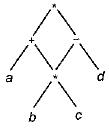Solution:

The above DAG represents
(a + b * C ) * { b * C - d )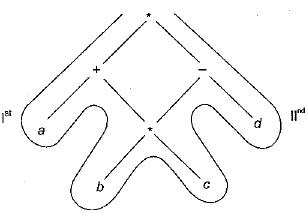QUESTION: 3

### Any Directed Acyclic Graph must have

Solution:

Simplest DAG: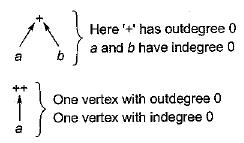QUESTION: 4

The DAG shown here represents: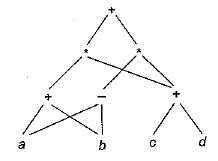Solution:

Simplifying the DAG: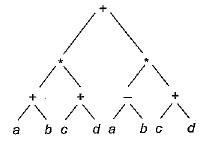Thus result: (a + b) * (c + cf) + (a - b) * (c + d)

QUESTION: 5

Consider the DAG with V = {1, 2, 3, 4, 5, 6}, shown below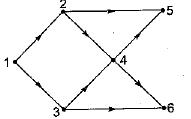Which of the following is NOT a topological ordering

Solution:
QUESTION: 6

A directed acyclic graph represents one form of intermediate representation. The number of non terminals nodes in DAG of a = (b + c)*(b + c) expression is

Solution: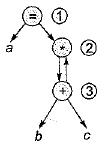Since indegree of node 3 is 1. So cannot start topological ordering with node 3 only start node will be 1.

QUESTION: 7

A pictorial representation of the value computed by each statement in the basic block is

Solution:

A pictorial representation of the value computed by. each statement in the basic block is control flow graph.

QUESTION: 8

DAG representation of a basic block allows

Solution:

DAG representation of a basic block allows automatic detection of local common sub expression.

QUESTION: 9

The intermediate code generated for the following syntax tree is: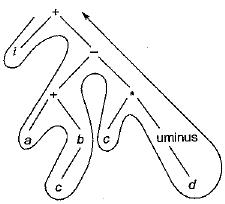Solution:

The intermediate code generated is iacb + cd uminus * - +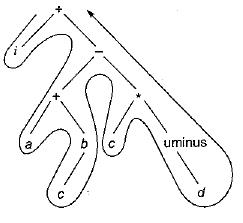QUESTION: 10

The triple representation for following representation is: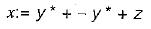Solution:
QUESTION: 11

Generation of intermediate code based on an abstract machine model is useful in compilers because

Solution:

Intermediate code enhance the portability of the front end of the compiler.

QUESTION: 12

Three address code’ technique for intermediate code generation shows that each statement usually contains three addresses. Three addresses are as follows:

Solution:

Three address code formate is two addresses for operands and one for operator.

QUESTION: 13

Consider the following arithmetic infix expression Q.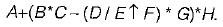Transform 'Q' into its equivalent postfix expression.

Solution:

Given expression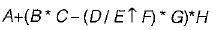Equivalent postfix expression;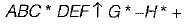QUESTION: 14

Which of the following is not a type of three address statements?

Solution:
QUESTION: 15

Consider the following statements:
S1: Three address code is a linearized representation of a syntax tree.
S2: The syntax tree not depicts the natural hierarchical structure of source program

Which of the above statements is/are true

Solution: